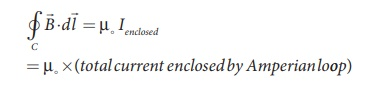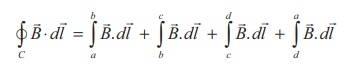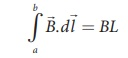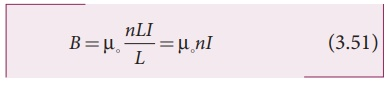Home | | Physics 12th Std | Magnetic field due to a long current carrying solenoid

# Magnetic field due to a long current carrying solenoid

Consider a solenoid of length L having N turns.

Magnetic field due to a long current carrying solenoid

Consider a solenoid of length L having N turns. The diametre of the solenoid is assumed to be much smaller when compared to its length and the coil is wound very closely.In order to calculate the magnetic field at any point inside the solenoid, we use Ampere’s circuital law. Consider a rectangular loop abcd as shown in Figure 3.46. Then from Ampère’s circuital law,The left hand side of the equation isSince the elemental lengths along bc and da are perpendicular to the magnetic field which is along the axis of the solenoid, the integralsSince the magnetic field outside the solenoid is zero, the integralFor the path along ab, the integral iswhere the length of the loop ab as shown in the Figure 3.46 is h. But the choice of length of the loop ab is arbitrary. We can take very large loop such that it is equal to the length of the solenoid L. Therefore the integral isLet N I be the current passing through the solenoid of N turns, thenThe number of turns per unit length is given by N/L = n, ThenSince n is a constant for a given solenoid and μ0 is also constant. For a fixed current I, the magnetic field inside the solenoid is also a constant.

EXAMPLE 3.19

Calculate the magnetic field inside a solenoid, when

(a) the length of the solenoid becomes twice and fixed number of turns

(b) both the length of the solenoid and number of turns are double

(c) the number of turns becomes twice for the fixed length of the solenoid

Compare the results.

Solution

The magnetic field of a solenoid (inside) is(a) length of the solenoid becomes twice and fixed number of turns

L→2L (length becomes twice)

N→N (number of turns are fixed)

The magnetic field is

B2L , N = µ NI/2L = 1/2 BL ,N

(b) both the length of the solenoid and number of turns are double

L→2L (length becomes twice)

N→2N (number of turns becomes twice)

The magnetic field is(c) the number of turns becomes twice but for the fixed length of the solenoid

L→L (length is fixed)

N→2N (number of turns becomes twice)

The magnetic field is

BL ,2 N = µ, 2NI/L = 2BL ,N

From the above results,

BL ,2 N > B2 L ,2 N > B2 L , N

Thus, strength of the magnetic field is increased when we pack more loops into the same length for a given current.

Tags : Ampere’s Circuital Law | Physics , 12th Physics : Magnetism and Magnetic Effects of Electric Current
Study Material, Lecturing Notes, Assignment, Reference, Wiki description explanation, brief detail
12th Physics : Magnetism and Magnetic Effects of Electric Current : Magnetic field due to a long current carrying solenoid | Ampere’s Circuital Law | Physics